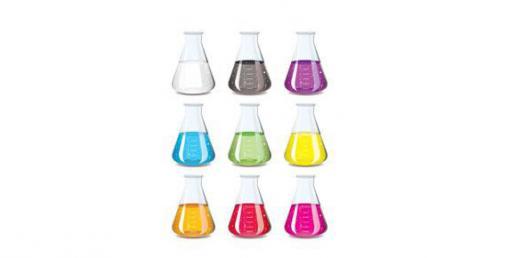# Chapter 7 Test: Chemical Equations

6 Questions | Attempts: 2821SettingsYou are going to take a quiz on chemical equations. Answer the following questions by selecting the correct choice.

• 1.
The products and the reactants in the reaction have the same ___.
• A.

Atoms

• B.

Molecules

• C.

Subscripts

• D.

Bond energies

• 2.
A balanced equation represents the law of mass conservation, and as a result all of the following are true except ___.
• A.

The number of moles of products equals the number of moles of reactants

• B.

The total mass of the product equals the total mass of the reactants

• C.

The number of atoms in the products equals the number of atoms in the reactants

• D.

The states of matter of the products are independent of the states of reactants

• 3.
A chemical reaction has not occurred if the products have ___.
• A.

The same mass as the reactants

• B.

Less total bond energy than the reactants

• C.

More total bond energy than the reactants

• D.

The same chemical properties as the reactants

• 4.
The chemical reaction is exothermic if ___.
• A.

∆H is a negative value

• B.

∆H is a positive value

• C.

Energy is absorbed during the reaction

• D.

Energy is conserved during reaction

• 5.
The only single-displacement reaction among the following chemical equations is ___.
• A.

2H2O2(l) → 2H2O(l) + O2(g)

• B.

Pb(s) + Hg2SO4(s) → PbSO4(s) + 2Hg(l)

• C.

2NH3(g) → N2(g) + 3H2(g)

• D.

NaCl(aq) + AgNO3(aq) → AgCl(s) + NaNO→3(aq)

• 6.
C(s) + O2(g) → CO2(g)+ energy is a chemical reaction because ____.
• A.

It releases energy

• B.

The chemical properties of CO2 are different from the individual properties of carbon and oxygen

• C.

It absorbs energy

• D.

CO2 is a gas and carbon is a crystal

## Related TopicsBack to top
×

Wait!
Here's an interesting quiz for you.